# Introduction

Let’s come directly on the point, in this article, we will try to develop a machine model on “Default of Credit Card Clients Dataset” hosted on Kaggle and precut whether a customer will default the payment next month.

# Dataset Information

This dataset contains information on default payments, demographic factors, credit data, history of payment, and bill statements of credit card clients in Taiwan from April 2005 to September 2005.

# Content

There are 25 variables:

• ID: ID of each client
• LIMIT_BAL: Amount of given credit in NT dollars (includes individual and family/supplementary credit
• SEX: Gender (1=male, 2=female)
• EDUCATION: (1=graduate school, 2=university, 3=high school, 4=others, 5=unknown, 6=unknown)
• MARRIAGE: Marital status (1=married, 2=single, 3=others)
• AGE: Age in years
• PAY_0: Repayment status in September 2005 (-1=pay duly, 1=payment delay for one month, 2=payment delay for two months, … 8=payment delay for eight months, 9=payment delay for nine months and above)
• PAY_2: Repayment status in August 2005 (scale same as above)
• PAY_3: Repayment status in July 2005 (scale same as above)
• PAY_4: Repayment status in June 2005 (scale same as above)
• PAY_5: Repayment status in May 2005 (scale same as above)
• PAY_6: Repayment status in April 2005 (scale same as above)
• BILL_AMT1: Amount of bill statement in September 2005 (NT dollar)
• BILL_AMT2: Amount of bill statement in August 2005 (NT dollar)
• BILL_AMT3: Amount of bill statement in July 2005 (NT dollar)
• BILL_AMT4: Amount of bill statement in June 2005 (NT dollar)
• BILL_AMT5: Amount of bill statement in May 2005 (NT dollar)
• BILL_AMT6: Amount of bill statement in April 2005 (NT dollar)
• PAY_AMT1: Amount of previous payment in September 2005 (NT dollar)
• PAY_AMT2: Amount of previous payment in August 2005 (NT dollar)
• PAY_AMT3: Amount of previous payment in July 2005 (NT dollar)
• PAY_AMT4: Amount of previous payment in June 2005 (NT dollar)
• PAY_AMT5: Amount of previous payment in May 2005 (NT dollar)
• PAY_AMT6: Amount of previous payment in April 2005 (NT dollar)
• default.payment.next.month: Default payment (1=yes, 0=no)

You have noticed that we have that 25 data features to deal with and we will mainly use the machine learning models:

• Logistic Regression
• Random Forest Classifier
• XGBoost Classifier

I hope that you have the data handy and let’s dive into the coding part,

## 1. Import Necessary Packages

At first, import the necessary packages that you think will help us for predicting results.

`import numpy as npimport pandas as pdimport matplotlib.pyplot as pltimport seaborn as snsimport osimport sys%matplotlib inlineimport warningswarnings.filterwarnings('ignore')`

`df = pd.read_csv('UCI_Credit_Card.csv')df.head()`

After loading the dataset, just have a look at the first five rows of the dataset. Now we try to execute some basic computations to understand the data.

`#Look at the number and name of columns in dataset.print(df.columns)print(df.shape)`
`#Check the info() of the dataset whether all the columns in dataset have the same datatype or not.df.info()`

That’s pretty great, we have columns of datatype int64 and float64 only. There is no object type data feature. Now let’s check whether our dataset has missing values or not.

`#Checking for missing valuesdf.isnull().sum()`

Ok! that’s even great we don’t have any missing values to handle.

# 3. Data Analysis

Let’s have a look at the target variable “default.payment.next.month” and distribution of that feature data.

`#Check the distribution of datadf['default.payment.next.month'].value_counts().plot.bar()`

From the above result, you can conclude that many of the clients are not interested in a payment next month.

Now let’s go through some quick data analysis and look at the distribution of data of the other data features.

`df['SEX'].value_counts().plot.bar()`

It finds that The number of Male credit holder is less than Female.

`sns.distplot(df['AGE'],kde=True,bins=30)`

There is a large number of clients whose age is between 25 to 40.

`df['EDUCATION'].value_counts().plot.bar()`

It looks like most of the client’s education level belongs to category 2,1 and 3.

`df['MARRIAGE'].value_counts().plot.bar()`

We noticed that there is very less number of values for category 3 and 0.

`sns.countplot(x='SEX', data=df,hue="default.payment.next.month", palette="muted")`

For females, the count of default.payment.next.month = 0 is highter than males.

`sns.countplot(x='EDUCATION',data=df,hue="default.payment.next.month",palette="muted")`
`sns.countplot(x='MARRIAGE',data=df,hue="default.payment.next.month", palette="muted")`

Almost there is an equal number of clients who default the payment next month for both the Married and Single category.

I would appreciate it if you go much deeper into the univariate and bivariate analysis. You can refer my code also for more data analytical approaches. The code is uploaded on GitHub.

Now let’s do some data pre-processing steps and find some interesting patterns in the dataset.

# 4. Data Processing

Let’s extract some insights for some data features if they want to tell us something. We will find it out.

`df['PAY_0'].value_counts()`

There is some double-digit count of values of the PAY_0 data feature and for some data features also. So we will create one single category of all low categories having less count.

`fill = (df.PAY_0 == 4) | (df.PAY_0==5) | (df.PAY_0==6) | (df.PAY_0==7) | (df.PAY_0==8)df.loc[fill,'PAY_0']=4df.PAY_0.value_counts()`

Let’s do it for the rest of the data features.

`fill = (df.PAY_2 == 4) | (df.PAY_2 == 1) | (df.PAY_2 == 5) | (df.PAY_2 == 7) | (df.PAY_2 == 6) | (df.PAY_2 == 8)df.loc[fill,'PAY_2']=4#df.PAY_2.value_counts()fill = (df.PAY_3 == 4) | (df.PAY_3 == 1) | (df.PAY_3 == 5) | (df.PAY_3 == 7) | (df.PAY_3 == 6) | (df.PAY_3 == 8)df.loc[fill,'PAY_3']=4#df.PAY_3.value_counts()fill = (df.PAY_4 == 4) | (df.PAY_4 == 1) | (df.PAY_4 == 5) | (df.PAY_4 == 7) | (df.PAY_4 == 6) | (df.PAY_4 == 8)df.loc[fill,'PAY_4']=4#df.PAY_4.value_counts()fill = (df.PAY_5 == 4) | (df.PAY_5 == 7) | (df.PAY_5 == 5) | (df.PAY_5 == 6) | (df.PAY_5 == 8)df.loc[fill,'PAY_5']=4#df.PAY_5.value_counts()fill = (df.PAY_6 == 4) | (df.PAY_6 == 7) | (df.PAY_6 == 5) | (df.PAY_6 == 6) | (df.PAY_6 == 8)df.loc[fill,'PAY_6']=4#df.PAY_6.value_counts()`

Now when you look at data there are some data features that have values on a large scale like bill_amt variables and many more. So we need to scale that variables.

`df.columns = df.columns.map(str.lower)col_to_norm = ['limit_bal', 'age', 'bill_amt1', 'bill_amt2', 'bill_amt3', 'bill_amt4', 'bill_amt5', 'bill_amt6', 'pay_amt1', 'pay_amt2', 'pay_amt3', 'pay_amt4', 'pay_amt5', 'pay_amt6']#you can inbuilt StandardScalar() or MinMaxScalar() alsodf[col_to_norm] = df[col_to_norm].apply(lambda x :( x-np.mean(x))/np.std(x))#df.head()`

Great! Feature scaling is done.

# 5. Correlation

Now we check the correlation of the independent variables with our target(dependent) variable.

`correlation = df.corr()plt.subplots(figsize=(30,10))sns.heatmap(correlation, square=True, annot=True, fmt=".1f" )`

Looking at the heatmap, you figured out that target variable default.payment.next.month depends on pay variables more. But I don’t suggest you drop the other features because it will be the loss of information. You can have a try of training the model with the most dependent features and evaluate the model also.

# 6. Predictive Modeling

Okk! now move towards predictive modeling. First, we split the training data into train and test using train_test_split().

`df = df.drop(["id"],1)X = df.iloc[:,:-1].valuesy = df.iloc[:,-1].values#We split the data into train(0.75) and test(0.25) size. from sklearn.model_selection import train_test_splitX_train,X_test,y_train,y_test = train_test_split(X,y,test_size = 0.25,random_state = 1)`

Let’s apply different machine learning models and evaluate the accuracy of the model.

• Logistic Regression Model
`#Start with logistic regression modelfrom sklearn.linear_model import LogisticRegressionlogmodel = LogisticRegression(random_state=1)logmodel.fit(X_train,y_train)y_pred = logmodel.predict(X_test)from sklearn.metrics import  accuracy_score, f1_score, precision_score, recall_score, roc_auc_scoreroc=roc_auc_score(y_test, y_pred)acc = accuracy_score(y_test, y_pred)prec = precision_score(y_test, y_pred)rec = recall_score(y_test, y_pred)f1 = f1_score(y_test, y_pred)results = pd.DataFrame([['Logistic Regression', acc,prec,rec, f1,roc]],columns = ['Model', 'Accuracy', 'Precision', 'Recall', 'F1 Score','ROC'])results`
`#plotting the confusion matrixfrom sklearn.metrics import confusion_matrixcm = confusion_matrix(y_test, y_pred)sns.heatmap(cm, cmap="Blues", annot=True,annot_kws={"size": 16})`

That’s a great attempt, we got the accuracy of 0.805467. Let’s apply some different models also,

• Random Forest Classifier Model
`#Apply Random Forest Classifierfrom sklearn.ensemble import RandomForestClassifierrfc = RandomForestClassifier(n_estimators = 100,criterion = 'entropy',random_state = 0)rfc.fit(X_train,y_train)y_pred = rfc.predict(X_test)roc=roc_auc_score(y_test, y_pred)acc = accuracy_score(y_test, y_pred)prec = precision_score(y_test, y_pred)rec = recall_score(y_test, y_pred)f1 = f1_score(y_test, y_pred)results = pd.DataFrame([['Random tree Classifier', acc,prec,rec, f1,roc]],columns = ['Model', 'Accuracy', 'Precision', 'Recall', 'F1 Score','ROC'])results`
`#plotting the confusion matrixfrom sklearn.metrics import confusion_matrixcm = confusion_matrix(y_test, y_pred)sns.heatmap(cm, cmap="Blues", annot=True,annot_kws={"size": 16})`

That’s a good improvement, let’s try with another model.

• XGBoost Classifier
`#Apply XGBoost classifier modelfrom xgboost import XGBClassifierxgb = XGBClassifier()xgb.fit(X_train, y_train)y_pred =xgb.predict(X_test)roc=roc_auc_score(y_test, y_pred)acc = accuracy_score(y_test, y_pred)prec = precision_score(y_test, y_pred)rec = recall_score(y_test, y_pred)f1 = f1_score(y_test, y_pred)results = pd.DataFrame([['XGBOOST Classifier', acc,prec,rec, f1,roc]],columns = ['Model', 'Accuracy', 'Precision', 'Recall', 'F1 Score','ROC'])results`
`#plotting the confusion matrixfrom sklearn.metrics import confusion_matrixcm = confusion_matrix(y_test, y_pred)sns.heatmap(cm, cmap="Blues", annot=True,annot_kws={"size": 16})`

Now you can evaluate that our XGBoost classifier model got a higher accuracy of 0.8196.

# Observations

Using the three classifier models, the accuracy we obtained is as follows:

• Logistic Regression: 0.8054
• Random Forest Classifier: 0.8173
• XGBoost Classifier: 0.8196

# Key Takeaways

• Apply more different classifier models and evaluate them.
• Perform feature engineering and train the model with more relevant features.

The code is available on Github.

--

--

--

## More from Amey Band

Love podcasts or audiobooks? Learn on the go with our new app.

## Indicators with Plotly## Analyzing Steam Reviews and Users Data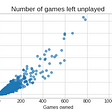## Sparkify Project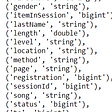## Exponential Growth and the R number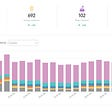## Starting off With ‘Google Data Studio’ via CXL Institute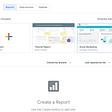## My hardest week yet as a Data Scientist. Ever heard of Machine Learning?## Flight Data Exploration Challenge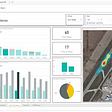## Explore Bagging Algorithm in Python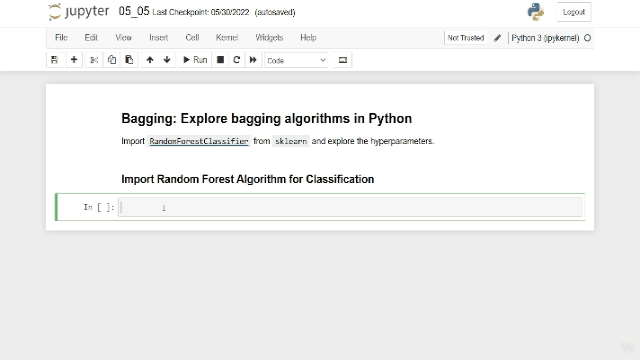## Gender Divide in Salaries- Capstone Project For Zero To Pandas Course## Machine Learning — Data Analysis